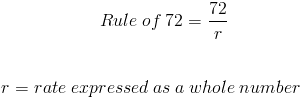# Rule of 72The Rule of 72 is a simple formula used to estimate the length of time required to double an investment. The rule of 72 is primarily used in off the cuff situations where an individual needs to make a quick calculation instead of working out the exact time it takes to double an investment. Also, one is more likely to remember the rule of 72 than the exact formula for doubling time or may not have access to a calculator that allows logarithms.

## Example of Rule of 72

An individual is earning 6% on their money market account would like to estimate how long it would take to double their current balance. In order for this estimation to be remotely accurate, we must assume that there will be no withdrawals nor deposits into this account. We can estimate that it will take approximately 12 years to double the current balance after dividing 72 by 6.

## Alternative Formula for Rule of 72

Alternatively, the rule of 72 can be applied to estimate the rate needed to double an investment within a specific time period. This alternative formula to the rule of 72 can be shown asIf we take a look at the prior example of Rule of 72, we can apply the same example to an individual wanting to estimate what their rate needs to be in order to double their money within a specific period of time. If an individual wants to estimate the rate needed to double their money within 12 years, this can be estimated as 6% from dividing 72 by 12 years.

## Breakdowns of Rule of 72

The rule of 72 is generally used for quick estimates in situations where the rate is in the several percent range. As the rate gets too low or too high below and above approximately 8%, the estimate becomes less accurate.

Another issue with the rule of 72 is with large sums of money. If a company or individual has large sums involved, this doesn't necessarily affect the outcome of the formula, but the company or individual may choose to use the actual doubling time formula as each decision could affect their profitability on a larger scale.

New to Finance?